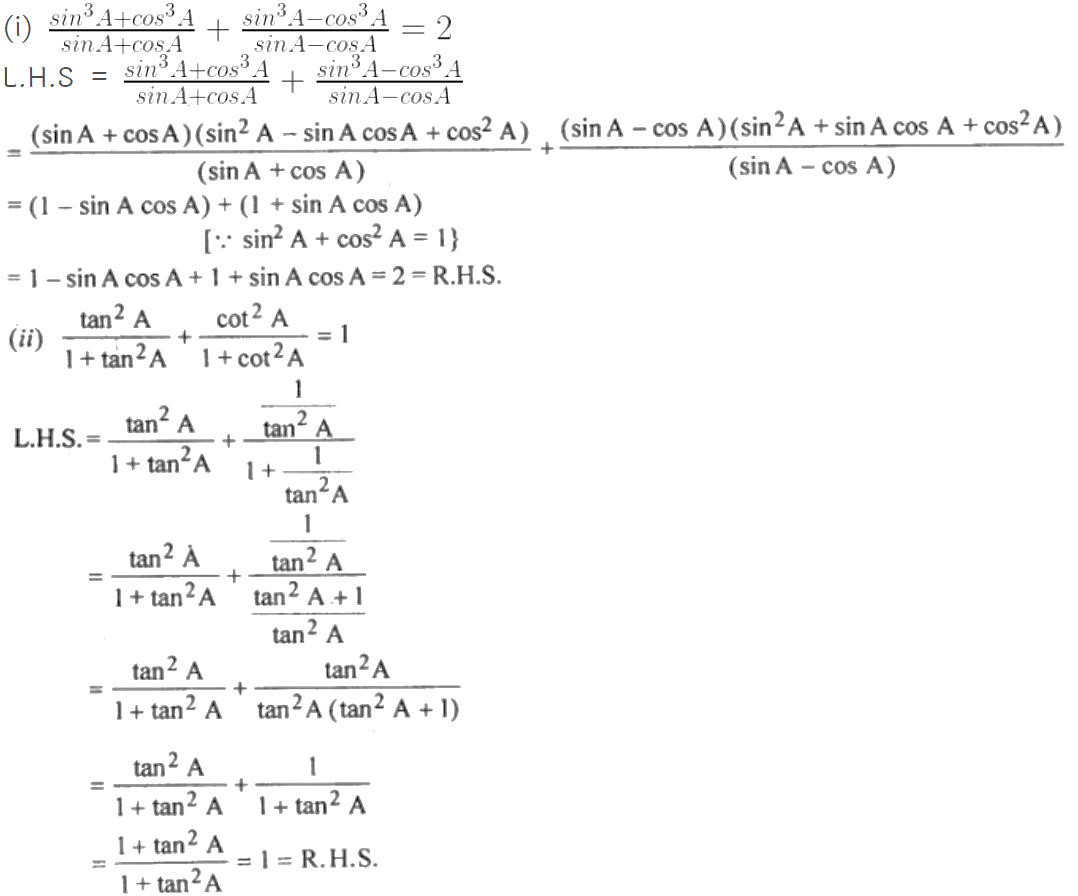Guru

# (i) Prove that (Sin^3A+cos^3A/sinA+cosA) + (sin^3A-cos^3A/sinA-cosA)=2 (ii)Prove that tan2A/(1+tan2A)+cot2A/(1+cot2A)=1

• 0

This is an important question from the
Book- ML Aggarwal
Board- ICSE
Publication- Avichal
Chapter- Trigonometric Identities
Chapter number-18

We have to prove the following Identities

(i) Prove that (Sin^3A+cos^3A/sinA+cosA) + (sin^3A-cos^3A/sinA-cosA)=2 (ii)Prove that tan2A/(1+tan2A)+cot2A/(1+cot2A)=1

Trigonometric Identities, class 10th, chapter 18th, question no29 , ICSE board, ML aggarwal..

Share